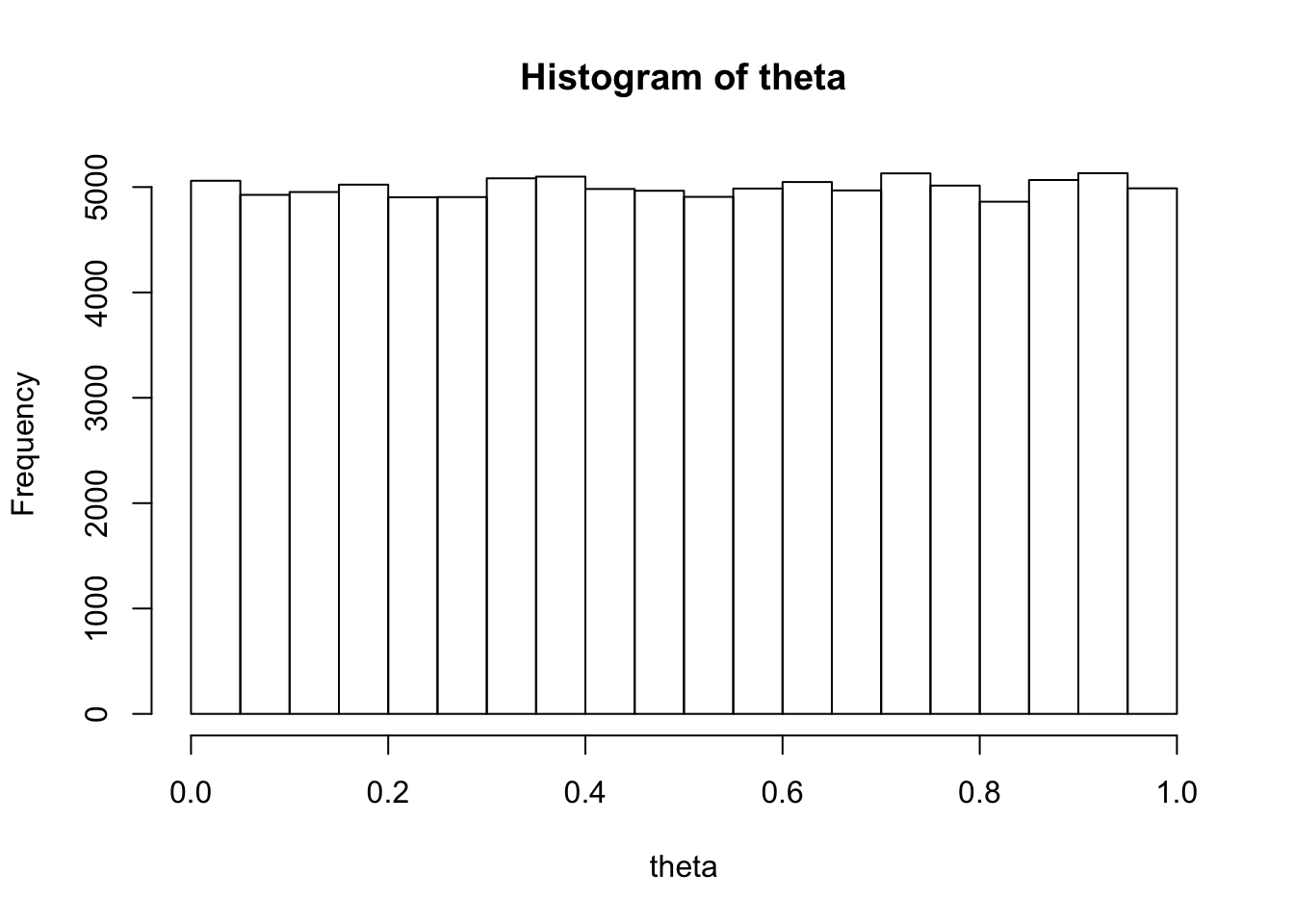### Abstract

When changing variables, a Jacobian adjustment needs to be provided to account for the rate of change of the transform. Applying the adjustment ensures that inferences that are based on expectations over the posterior are invariant under reparameterizations. In contrast, the posterior mode changes as a result of the reparameterization. In this note, we use Stan to code a repeated binary trial model parameterized by chance of success, along with its reparameterization in terms of log odds in order to demonstrate the effect of the Jacobian adjustment on the Bayesian posterior and the posterior mode. We contrast the posterior mode to the maximum likelihood estimate, which, like the Bayesian estimates, is invariant under reparameterization. Along the way, we derive the logistic distribution by transforming a uniformly distributed variable.

## Visualizing a Change of Variables

Suppose $$\theta \in (0,1)$$ represents a probability of success. Then the odds ratio is $$\frac{\theta}{1 - \theta} \in (0, \infty)$$. For example, if $$\theta = 0.75$$, the odds ratio is $$\frac{0.75}{1 - 0.75} = 3$$, whereas if $$\theta = 0.25$$, the odds ratio is $$\frac{0.25}{1 - 0.25} = \frac{1}{3}$$. An odds ratio of $$3$$ is conventionally written as $$3{:}1$$ and pronounced as “three to one odds” (in favor of success), wheras an odds ratio of $$\frac{1}{3}$$ is written as $$1{:}3$$ and prononced “one to three odds” (in favor, or “three to one odds against”). If $$\theta = 0.5$$, then the odds are $$1{:}1$$, or “even”.

The log odds is just the logarithm of the odds, and the function $$\mathrm{logit}:(0,1) \rightarrow \mathbb{R}$$ converts chance of success to log odds by $\mathrm{logit}(\theta) \ = \ \log \frac{\theta}{1 - \theta}.$ The nice thing about log odds is that they are symmetric around zero. If the chance of success is $$0.5$$, the odds ratio is $$1$$, and the log odds are $$0$$. If the chance of success is $$0.75$$ then the log odds are just $$\log 3$$, or about $$1.10$$, whereas if the chance of success is $$0.25$$, the log odds are just $$\log \frac{1}{3} = -\log 3$$, or about $$-1.10$$. The log-odds transform has the symmetry $\mathrm{logit}(\theta) \ = \ -\mathrm{logit}(1 - \theta),$ which is useful for defining symmetric priors on log odds centered around zero.

Now suppose we have a uniformly-distributed random variable $$\theta \sim \mathsf{Uniform}(0,1)$$ representing a chance of success. We can draw a large sample and plot a histogram.

theta <- runif(100000, 0, 1);
hist(theta, breaks=20);It’s clear this is a nice uniform distribution. Now what happens if we log-odds transform the draws?

logit <- function(u) log(u / (1 - u));
logit_theta <- logit(theta);
hist(logit_theta, breaks=20);